Home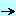papersoop_componentsdelphi_generics_tutorial

## Delphi .Net Generics Tutorial - Felix John COLIBRI.

• abstract : using Generics (parameterized types) in Delphi : the type parameter and the type argument, application of generics, constraints about INTERFACEs or CONSTRUCTORs
• key words : generics, parameterized types, constraints, genericity
• software used : Windows XP Home, Rad Studio 2007 (Delphi 2007 for .Net)
• hardware used : Pentium 2.800Mhz, 512 M memory, 140 G hard disc
• scope : Rad Studio 2007 .Net, Delphi 2008 Win32 (to come)
• level : Delphi developer
• plan :

## 1 - Why Generics ?

Generics (also called Parameterized types or Generic types) allow us to write more general code, while keeping type safety.

Delphi tList, tStringList, tObjectlist or tCollection can be used to build specialized containers, but require type casting. With Generics, casting is avoided and the compiler can spot type errors sooner.

## 2 - Life Before Generics

### 2.1 - Our basic example: a stack

We will illustrate the difference between usual techniques and the use of Generics with the classical bounded stack example: we want to store data on a fixed size stack using Push and Pop.

### 2.2 - An Integer stack

We create the stack with this simple CLASS:

 unit u_c_genc_101_integer_stack;   interface     type c_integer_stack= Class                             m_integer_array: Array of Integer;                             m_top_of_stack: Integer;                             constructor create_integer_stack(p_length: Integer);                             procedure push(p_integer: Integer);                             function f_pop: Integer;                           end; // c_integer_stack   implementation     // -- c_integer_stack     constructor c_integer_stack.create_integer_stack(p_length: Integer);       begin         Inherited Create;         SetLength(m_integer_array, p_length);       end; // create_integer_stack     procedure c_integer_stack.push(p_integer: Integer);       begin         if m_top_of_stack< Length(m_integer_array)           then begin               m_integer_array[m_top_of_stack]:= p_integer;               Inc(m_top_of_stack);             end;       end; // push     function c_integer_stack.f_pop: Integer;       begin         if m_top_of_stack>= 0           then begin               Dec(m_top_of_stack);               Result:= m_integer_array[m_top_of_stack];             end           else raise Exception.Create('empty') ;       end; // f_pop   end.

and use it like this:

 program p_genc_001_array_of_integer;   uses   SysUtils,   u_c_genc_101_integer_stack in 'u_c_genc_101_integer_stack.pas';   var g_c_integer_stack: c_integer_stack;   begin // main     g_c_integer_stack:= c_integer_stack.create_integer_stack(5);     with g_c_integer_stack do     begin       push(111);       push(222);       push(333);       // -- refused by the compiler:       // push('allistair');       writeln(f_pop);       writeln(f_pop);       writeln(f_pop);     end; // with g_c_integer_stack   end. // main

Note that

• since the compiler knows that we declared an ARRAY OF Integer, it immediately refuses to push any type incompatible with Integers, which is the case for Strings
• we can build an Stack of Strings, but we then have two separate units which have to evolve and to be maintained separately

### 2.3 - A stack of tObject with type casting

We can generalize the previous code by using an ARRAY OF tObject:
• the CLASS only uses the tObject type
• the assignment rules of Delphi allows us to push any tObject or any of its descendents, like a c_person object
• to retrieve the values, we MUST use type casting
Here is our tObject CLASS:

 unit u_c_object_stack;   interface     type c_object_stack= class                            m_object_array: Array of tObject;                            m_top_of_stack: Integer;                            constructor create_object_stack(p_length: Integer);                            procedure push(p_c_object: tObject);                            function f_pop: tObject;                          end; // c_object_stack   implementation     uses SysUtils;     // -- c_object_stack     constructor c_object_stack.create_object_stack(p_length: Integer);       begin         Inherited Create;         SetLength(m_object_array, p_length);       end; // create_object_stack     procedure c_object_stack.push(p_c_object: tObject);       begin         if m_top_of_stack< Length(m_object_array)           then begin               m_object_array[m_top_of_stack]:= p_c_object;               Inc(m_top_of_stack);             end;       end; // push     function c_object_stack.f_pop: tObject;       begin         if m_top_of_stack>= 0           then begin               Dec(m_top_of_stack);               Result:= m_object_array[m_top_of_stack];             end           else raise Exception.Create('empty') ;       end; // f_pop     end. // u_c_object_stack

Here are an examples of storing

• Integers:

 procedure use_integer_stack;   var l_c_integer_stack: c_object_stack;   begin     l_c_integer_stack:= c_object_stack.create_object_stack(5);     with l_c_integer_stack do     begin       push(tObject(111));       push(tObject(222));       writeln(f_pop);       writeln(f_pop);     end; // with l_c_integer_stack   end; // use_integer_stack

• or custom c_person objects:

 // -- c_person type c_person= Class                  m_first_name: String;                  Constructor create_person(p_first_name: String);                end; // c_person constructor c_person.create_person(p_first_name: String);   begin     Inherited Create;     m_first_name:= p_first_name;   end; // createp_person // -- c_person stack procedure use_person_stack;   var l_c_person_stack: c_object_stack;   begin     l_c_person_stack:= c_object_stack.create_object_stack(5);     with l_c_person_stack do     begin       push(c_person.create_person('ann'));       // -- accepted here       push(tObject(222));       push(c_person.create_person('allistair'));       writeln(c_person(f_pop).m_first_name);       // -- poping an Integer as a c_person will fail here       writeln(c_person(f_pop).m_first_name);       writeln(c_person(f_pop).m_first_name);     end; // with l_c_person_stack   end; // use_person_stack

Note that
• for the Integer stack, we had to cast the Integer value into a tObject. This transformation of a Integer litteral into a CLASS in the Win32 world is simply a notation to force the compiler to consider the Integer as a tObject. No transformation takes place. The tObject is a 4 byte pointer, and the Integer a 4 byte value. In the .Net world, things are quite different: 123 is a litteral and a VAR my_integer with value 123 is a CLASS, which has methods, like ToString. We can write my_integer.ToString, we cannot write 123.ToString. The transformation of the litteral into a CLASS is called boxing and is not free: the compiler must generate code for the conversion
• the Writeln accepted to print the value of a tObject as an Integer value. Therefore casting is not mandatory to print the f_pop of the Integer
• for the c_person stack
• casting was not necessary for the assignment (c_person is "assignment compatible" with tObject
• casting was necessary to retrieve the top of stack as a c_person
• if we cast an Integer as a c_person, there is an exception AT RUNTIME. Thats exactly the problem: when we use casting, we force the compiler to believe that the object if of some type, and this will be blindly accepted at compile time. When we cast a wrong object, the error is detected later
• any cell can contain any tObject descendent. So this structure is polymorphic: we can store objects of different types in the same stack, but the retrieval will necessitate a lot of testing to extract the correct type (using IS or AS)

### 2.4 - A .Net Collection

The previous examples were written using the Win32 personality of Delphi. We face the same problems with the .Net personality: either we write type safe code tied to a specific type, or we use casting, with the risk of encountering run time exception.

Here is an example:

 program p_genc_003_array_of_object;   uses System.Collections;   type c_person= Class                    m_first_name: String;                    Constructor create_person(p_first_name: String);                  end; // c_person   // -- c_person   constructor c_person.create_person(p_first_name: String);     begin       Inherited Create;       m_first_name:= p_first_name;     end; // createp_person   procedure use_person_arraylist;     var l_c_person_list: ArrayList;         l_c_person: c_person;     begin       l_c_person_list:= ArrayList.Create;       l_c_person_list.Add(c_person.create_person('Miller'));       l_c_person_list.Add(c_person.create_person('Smith'));       // -- this is accepted       l_c_person_list.Add('xxx');       // -- the 'xxx' entry will trigger an exception       for l_c_person in l_c_person_list do         writeln(l_c_person.m_first_name);     end; // use_person_arraylist   begin // main     use_person_arraylist;     writeln; write('=> type enter'); Readln;   end. // main

Note that:

• we can assign the objects without type casting, since c_person is an Object descendent
• the FOR IN construct apparently does not require casting. In fact the compiler does the casting for us, and we still get a run time exception if an object is not of the expected type

## 3 - The First Generics Example

### 3.1 - Our first Generic example

To create a generic stack
• in the CLASS definition:
• we add a type parameter between "<" and ">" just after the CLASS name

 type c_generic_stack= class        // ---ooo---

• this T parameter can be placed at the same places as any TYPE: in member fields, in CONSTRUCTOR's or method's parameters, in FUNCTION's result type:

 type c_generic_stack= class                            m_gen_array: Array of T;                            m_top_of_stack: Integer;                            constructor create_generic_stack(p_length: Integer);                            procedure push(p_gen: T);                            function f_pop: T;                          end; // c_generic_stack

• in the IMPLEMENTATION, we use the identifiers of type T:
• we add the type parameter <T> after each method name. For instance:

 procedure c_generic_stack.push(p_gen: T);   begin     // --ooo--

• and we use any parameter or variables of type T in our code:

 procedure c_generic_stack.push(p_gen: T);   begin     if m_top_of_stack< Length(m_gen_array)       then begin           m_gen_array[m_top_of_stack]:= p_gen;           Inc(m_top_of_stack);         end;   end; // push

• in order to use this CLASS, we must specify which concrete kind of objects we want to use in each cell of our stack
• at the VAR declaration level, we write the concrete type between "<" and ">":

 var my_c_integer_stack: c_generic_stack;

• this type is also repeated during the CONSTRUCTOR call:

 my_c_integer_stack:= c_generic_stack.create_generic_stack(5); my_c_integer_stack.push(111); writeln(my_c_integer_stack.f_pop);

Here is the complete example:
• the CLASS definition:

 unit u_c_genc_020_generic_stack;   interface     type c_generic_stack= class                                m_gen_array: Array of T;                                m_top_of_stack: Integer;                                constructor create_generic_stack(p_length: Integer);                                procedure push(p_gen: T);                                function f_pop: T;                              end; // c_generic_stack   implementation     // -- c_generic_stack     constructor c_generic_stack.create_generic_stack(p_length: Integer);       begin         Inherited Create;         SetLength(m_gen_array, p_length);       end; // create_generic_stack     procedure c_generic_stack.push(p_gen: T);       begin         if m_top_of_stack< Length(m_gen_array)           then begin               m_gen_array[m_top_of_stack]:= p_gen;               Inc(m_top_of_stack);             end;       end; // push     function c_generic_stack.f_pop: T;       begin         if m_top_of_stack>= 0           then begin               Dec(m_top_of_stack);               Result:= m_gen_array[m_top_of_stack];             end           else raise Exception.Create('empty') ;       end; // f_pop     end. // u_c_generic_stack

• used for an Integer stack:

 procedure use_integer_stack;   var l_c_integer_stack: c_generic_stack;   begin     l_c_integer_stack:= c_generic_stack.create_generic_stack(5);     with l_c_integer_stack do     begin       push(111);       push(222);       // -- refused by the compiler       // push('Abigail');       writeln(f_pop);       writeln(f_pop);     end; // with l_c_integer_stack   end; // use_integer_stack

• or for a c_person stack :

 // -- c_person type c_person= Class                  m_first_name: String;                  Constructor create_person(p_first_name: String);                end; // c_person constructor c_person.create_person(p_first_name: String);   begin     Inherited Create;     m_first_name:= p_first_name;   end; // createp_person // -- a c_person stack procedure use_person_stack;   var l_c_person_stack: c_generic_stack;   begin     l_c_person_stack:= c_generic_stack.create_generic_stack(5);     with l_c_person_stack do     begin       push(c_person.create_person('ann'));       push(c_person.create_person('allistair'));       // -- refused by the compiler       // Push(111);       writeln(f_pop.m_first_name);       writeln(f_pop.m_first_name);     end; // with l_c_person_stack   end; // use_person_stack

### 3.2 - Terminology

Just a couple of naming conventions:
• the identifier located after the TYPE name (T in our case) is called the "type parameter" (or sometimes the "formal type parameter")
• the real concrete type that we give when we want to declare a VAR or a parameter (Integer in our case), is called the "type argument" (or sometimes the "actual parameter")
• c_stack<t_gen> is an open constructed type, and c_stack<Integer> a closed constructed type

• the identifier of the type parameter is indifferent: any Pascal identifier is acceptable. For very general CLASSes, it is often a single letter, like T, but using longer names, like measure or content is also correct.

 type c_currency= Class                        end;      c_dictionary= Class                                end;      c_calculator= Class                                          end;

For long names, we will use a convention where the type parameter starts with an upper case T_ followed by some meaningfull name, like T_measure

• the compiler rejects the definition of a second CLASS with the same name but with parameter types having a different name only. It is possible, as will be shown later, to uses several parameter types. If the "parameter signature" is different, then we can use the same CLASS name

 type c_stack= Class                      end;      // NO : c_stack= Class      c_stack= Class                                 end;

### 3.3 - How are Generics implemented ?

Generics are implemented at the .Net intermediate language level (IL: Intermediate Language= C# pseudo code) and the CLR level (Common Language Runtime: the library managing the code, containing, the IL-to-native compiler, the type checker, the loader, the memory manager etc).

The intermediate language contains :

• the parameterized types, along with the standard types
• markers for the type arguments
• informations about generics included in the IL meta data
When the intermeditate code is compiled into binary code (x386 assembler)
• when the code defines type arguments, the metadata is used to update the generic metatdata with the argument metadata
• the compiler can then perform its type checking
• if the type argument is a value type (Integer, Double etc), the parameters are replaced with the actual type, and the corresponding code is generated. Therefore, there is no boxing / unboxing for those actual types. In addition, if the type is used in some other places, the compiler uses a reference pointing to the compiled code
• if the type argument is a reference type (classe, arrays, lists etc), the type parameter is replaced by tObject. The native code uses a reference pointing to the object, and this without any casting.

## 4 - Using Parameterized Types

### 4.1 - Generic CLASSes

As presented before, a CLASS can use a generic parameter

### 4.2 - Generic RECORDs

It is also possible to parameterize RECORDs. Here is an example:

 program p_genc_032_record;   uses SysUtils;   type t_point= Record                                 m_x, m_y, m_z: T_coordinate;                               end; // t_point   var g_center: t_point;       g_projection: t_point;   begin // main     g_center.m_x:= 100;     g_projection.m_x:= 3.1415;     writeln(g_center.m_x);     writeln(g_projection.m_x);   end. // main

### 4.3 - Generic ARRAYs

The cell type of an ARRAY can use parameters:

 program p_genc_032_array;   uses SysUtils;   type t_array= Array of T_cell;        t_xy_array= Array of Array of T_coordinate;        t_average_array= Array[1..5] of T_value;   var g_counts: t_array;       g_measures: t_array;       g_index: integer;       g_xy_array: t_xy_array;       g_array_of_double: Array of Double;   begin // main     SetLength(g_counts, 100);     for g_index:= 0 to 99 do       g_counts[g_index]:= Random(100);     SetLength(g_measures, 20);     for g_index:= 0 to 19 do       g_measures[g_index]:= 3.14* Random;     SetLength(g_xy_array, 10, 20);     g_xy_array[2, 3]:= 3.14;     SetLength(g_array_of_double, 10* 20);     g_array_of_double[2* 10+ 3]:= g_xy_array[2, 3];   end. // main

In the previous example, we showed

• two dimensional ARRAYs
• an example of mixing parameterized ARRAYs and usual ARRAYs

### 4.4 - Generic Procedural Types

We can use parameter types in the definition of Procedural Types.

The definition of some binary operator could be:

 unit u_genc_034_procedural_type;   interface     Type t_pg_handle_two= Procedure(p_one, p_two: T);   implementation     end. // u_genc_034_procedural_type

and we could apply this operator to Integers or Doubles:

 procedure convert_two_integer(p_value, p_rate: Integer);   begin     writeln(p_value, ' div ', p_rate, ' =', p_value Div p_rate);   end; // convert_two_integer procedure convert_two_double(p_value, p_rate: Double);   begin     writeln(p_value, ' / ', p_rate, ' =', p_value / p_rate);   end; // convert_two_double procedure use_binary_operator;   var l_pg_convert_two_integer: t_pg_handle_two;       l_pg_convert_two_double: t_pg_handle_two;   begin     l_pg_convert_two_integer:= convert_two_integer;     l_pg_convert_two_integer(20, 3);     l_pg_convert_two_double:= convert_two_double;     l_pg_convert_two_double(20.0, 3.0);   end; // use_binary_operator

And you will notice that the Integer division uses DIV whereas the Double division requires /.

Procedural types are usually employed to apply some handling to a set of values, and give some kind of "Lisp applicative" flavor to our programs. We define generic handling routines, and can specify AT RUNTIME, which concrete procedure will be applied.

Here is an example of a list of values with a generic handling routine:

 unit u_genc_035_apply_procedural_type;   interface     Type t_fg_handle_one= Function(p_value: T): T;          c_vector= Class                         m_vector: Array[0..9] of T;                         constructor Create;                         procedure compute(p_fg_handle_one: t_fg_handle_one );                         procedure display_vector;                       end;   implementation     uses SysUtils;     // -- c_vector     constructor c_vector.Create;       begin         Inherited;       end; // Create     procedure c_vector.compute(p_fg_handle_one: t_fg_handle_one);       var l_index: Integer;       begin         for l_index:= 0 to 9 do           m_vector[l_index]:= p_fg_handle_one(m_vector[l_index]);       end; // compute     procedure c_vector.display_vector;       var l_index: Integer;           l_result: String;       begin         l_result:= '';         for l_index:= 0 to 9 do           l_result:= l_result+ m_vector[l_index].ToString+ ' ';         writeln(l_result);       end; // display_vector     end. // u_genc_034_procedural_type

and we can use it like this:

 function f_integer_square(p_value: Integer): Integer;   begin     Result:= p_value* p_value;   end; // f_integer_square procedure use_vector;   var l_c_vector: c_vector;       l_index: Integer;   begin     l_c_vector:= c_vector.Create;     for l_index:= 0 to 9 do       l_c_vector.m_vector[l_index]:= l_index;     l_c_vector.display_vector;     l_c_vector.compute(f_integer_square);     l_c_vector.display_vector;   end; // l_c_vector

Note that:

• this is the first case where we start "combining" the generic elements
• to display the values, we could call Writeln(my_T_instance.ToString) since the compiler assumes that any type T has a ToString function

### 4.5 - Generic Events (PROCEDURE OF OBJECT)

Events are very similar to procedural type, but can only be defined in CLASSes. The inner working is the same, but the compiler simply pushes an additional transparent parameter which is the object which called the procedure. In addition, Events are more used to acknowledge the user of a CLASS that something is happening rather than apply some functional computation. They are in general used as some kind of callback (the mouse has been clicked, a character arrived from the network etc).

We define here a The definition of a PROCEDURE OF OBJECT which will notify us about any changes:

 unit u_genc_035_procedure_of_object;   interface     type t_po_notify_change= Procedure(p_value: T) Of Object;          c_storage= Class                          m_table: array of V;                          m_on_notify_value_changed: t_po_notify_change;                          m_on_notify_storage_changed: t_po_notify_change< c_storage >;                          constructor create_storage(p_size: Integer);                          procedure add_value(p_index: Integer; p_value: V);                        end; // c_storage   implementation     // -- Type c_storage     constructor c_storage.create_storage(p_size: Integer);       begin         Inherited Create;         SetLength(m_table, p_size);       end; // create_storage     procedure c_storage.add_value(p_index: Integer; p_value: V);       begin         m_table[p_index]:= p_value;         if Assigned(m_on_notify_value_changed)           then m_on_notify_value_changed(p_value);         if Assigned(m_on_notify_storage_changed)           then m_on_notify_storage_changed(Self);       end; // add_value     end. // u_genc_035_procedure_of_object

and we can use the event like this:

 program p_genc_035_procedure_of_object;   uses SysUtils,     u_genc_035_procedure_of_object in 'u_genc_035_procedure_of_object.pas';   Type c_statistics= Class                        m_c_storage: c_storage;                        constructor create_statistics;                        procedure display_value_changed(p_value: Integer);                        procedure display_storage_changed(p_c_storage: c_storage);                      end; // c_storage   // -- Type c_statistics   constructor c_statistics.create_statistics;     begin       Inherited Create;       m_c_storage:= c_storage.create_storage(5);       m_c_storage.m_on_notify_value_changed:= display_value_changed;       m_c_storage.m_on_notify_storage_changed:= display_storage_changed;     end; // create_statistics   procedure c_statistics.display_value_changed(p_value: Integer);     begin       Writeln('added_value ', p_value);     end; // display_value_changes   procedure c_statistics.display_storage_changed(p_c_storage: c_storage);     var l_index: Integer;     begin       Writeln('added_value_to ');       for l_index:= 0 to Length(p_c_storage.m_table)- 1 do         Writeln('  ', p_c_storage.m_table[l_index]);     end; // display_storage_changed   var g_c_statistics: c_statistics;   begin // main     g_c_statistics:= c_statistics.create_statistics;     g_c_statistics.m_c_storage.add_value(0, 33);   end. // main

Note that

• since events must be nested inside CLASSes, we had to create one for our example (c_statistics). In standard events handling, it is the tForm which harbors the delegated events like tButtonClick

### 4.6 - Generic Methods

We can generalize the methods of a CLASS:

 unit u_c_genc_36_generic_method;   interface     type c_product= class                       m_price: Double;                       m_quantity: Integer;                       Constructor create_product(p_price: Double; p_quantity: Integer);                       procedure display(p_text: String; p_value: T);                     end; // c_product   implementation     // -- c_product     Constructor c_product.create_product(p_price: Double; p_quantity: Integer);       begin         Inherited Create;         m_price:= p_price; m_quantity:= p_quantity;       end; // create_product     procedure c_product.display(p_text: String; p_value: T);       begin         writeln(p_text, p_value.ToString);       end; // display     end. // u_c_genc_36_generic_method

and we use it here:

 procedure use_generic_method;   var l_c_product: c_product;   begin     l_c_product:= c_product.create_product(218.15, 34);     l_c_product.display( 'the price is    ', l_c_product.m_price);     l_c_product.display('the quantity is ', l_c_product.m_quantity);   end; // use_generic_method

We can also have parameterized CLASS methods. Those methods can be called by directly calling the CLASS method, without having to create an object.

First, here is the CLASS

 unit u_c_genc_36_generic_class_method;   interface     type c_sort= Class                    Class procedure swap(Var pv_one, pv_two: T); Static;                  end; // c_sort   implementation     // -- c_sort     Class procedure c_sort.swap(Var pv_one, pv_two: T);       var l_temporary: T;       begin         l_temporary:= pv_two;         pv_two:= pv_one;         pv_one:= l_temporary       end; // swap     end. // u_c_genc_36_generic_class_method

and an example of how to use this swap CLASS method:

 procedure use_generic_class_method;   var l_entier_1, l_entier_2: Integer;       l_double_1, l_double_2: Double;   begin     l_entier_1:= 10; l_entier_2:= 200;     writeln('original ', l_entier_1: 5, l_entier_2: 5);     c_sort.swap(l_entier_2, l_entier_1);     writeln('    swap ', l_entier_1: 5, l_entier_2: 5);     writeln;     l_double_1:= 1.11; l_double_2:= 333.33;     writeln('original ', l_double_1: 7: 2, ' ', l_double_2: 7: 2);     c_sort.swap(l_double_1, l_double_2);     writeln('    swap ', l_double_1: 7: 2, ' ', l_double_2: 7: 2);   end; // use_generic_class_method

Note that

• the classical CLASS method in Delphi is the CONSTRUCTOR which is called using the c_class.Create typical syntax
• in the .Net world, the CLASS methods are much more important. C# is a Java clone, and in Java, everything is a CLASS. There are no global PROCEDUREs or FUNCTIONs: they necessarily belong to some CLASS. So if you want to compute a Sine, you have to nest this in some CLASS. This has then been pushed to the extreme, where the CLASS is used as a basic encapsulation mechanism. In .Net, the System.IO.File is a CLASS containing ONLY CLASS methods, like File.Copy. In Dbx4, many elements are definined in a CLASS. For instance the TDBXDataTypes.DateType is a CONST defined in a CLASS, and CLASS methods are used in many places, for factories (TDBXConnectionFactory.OpenConnectionFactory) or to get values which do not use the CLASS attributes (TDBXValueTypeEx.DataTypeName).

### 4.7 - Generic INTERFACEs

In addition to CLASSes, INTERFACEs can also use generics. A simple visitor could be defined with:

 unit u_i_genc_037_generic_interface;   interface     type i_visitor= Interface                               procedure Visit(p_node: T_node);                             end; // i_visitor          c_point= Class                             m_x, m_y: T_data;                             constructor create_point(p_x, p_y: T_data);                             function ToString: String; Override;                             procedure Accept(p_c_visitor: i_visitor< c_point > );                           end; // c_point          c_display_visitor= Class(tObject, i_visitor)                                       procedure Visit(p_node: T_node);                                     end; // c_display_visitor   implementation     // -- c_point     constructor c_point.create_point(p_x, p_y: T_data);       begin         Inherited Create;         m_x:= p_x; m_y:= p_y;       end; // create_point     procedure c_point.Accept(p_c_visitor: i_visitor< c_point > );       begin         p_c_visitor.Visit(Self);       end; // Accept     function c_point.ToString: String;       begin         writeln('x=', m_x.ToString+ ', y=' + m_y.ToString);       end; // ToString      // -- c_display_visitor      procedure c_display_visitor.Visit(p_node: T_node);        begin          writeln(p_node.ToString);        end; // Visit     end. // u_i_genc_037_generic_interface_5

and here is an example using this visitor :

 program p_genc_037_generic_interface;   uses SysUtils,       u_i_genc_037_generic_interface in 'u_i_genc_037_generic_interface.pas';   var g_c_point: c_point;       g_c_display_visitor: c_display_visitor< c_point >;   begin // main     g_c_point:= c_point.create_point(3, 50);     g_c_display_visitor:= c_display_visitor< c_point >.Create;     g_c_point.Accept(g_c_display_visitor);   end. // main  end. // main

Note that

• this is a very downgraded "visitor". In the classical Visitor design pattern, we usually have
• a structure (a document built from different elements, all descending from the same basic abstract CLASS)
• several visitors which "visit" each element of the structure, performing different specific tasks
The UM Class Diagram of such a bona fide visitor would be: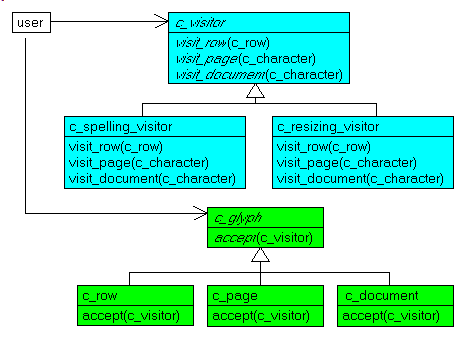Nevertheless, the possibility to use generic types for the visitor INTERFACE is a very nice improvement.

• generic types are very often associated with Design Patterns. This is not a surprise, since one of the basic mechanism of Design Patterns is to define an INTERFACE, and let the user select at RUNTIME any descendent which implements this INTERFACE.

• generic INTERFACEs are also often used with CONSTRAINTs, which are our next topic.

## 5 - Constraints on Generic Types

### 5.1 - Operations on Generic Types

At first glance, generics look like a technique of choice for building numerical libraries: computing averages, min values, max values, series handling, matrix computations.

This is not directly possible, and the reason is easy to understand: when we declare an ARRAY OF T, the compiler has no information whatsoever about T. Therefore it cannot generate any code for adding, comparing, multiplying T values. A TYPE specifies the possible values and the allowed operators:

• for Integers, values are numbers without fractional part: 3, -15, 0, and operators +, -, * ,DIV
• for Reals, values are numeric values with fractional part: 3.14, -12.23e-18, and operators +, -, *, /
Now when the compilers sees T, there is no indication as to what the future argument will be. It could be Integer (with +, *, DIV), Double (with +, -, but / and not DIV), String (with only +) or any CLASS (c_person, with no arithmetic operation whatsoever).

The bottom line is that in order to perform some meaningfull handling on the data of type T, we must tell the compiler which operations will be available for the T concrete arguments. We somehow reduce the very general type T to some type subset, where, for instance, addition, or comparison are possible. We impose constraints on the type parameter T.

What kind of constraints ? Currently, we can constraint T

• to implement some INTERFACE
• to be a CLASS (as opposed to be value type)
• to be a descendent of some specific CLASS
• to have a default parameterless CONSTRUCTOR

### 5.2 - INTERFACE constraint

We will first start with the simple = operator. We want to be able to locate a value in a structure. So we must be able to tell whether two values are the same. In the .Net world, this is defined by the iEquatable INTERFACE, defined in the .Net Help (.Net Framework SDK | Class Library | System | iEquatable) :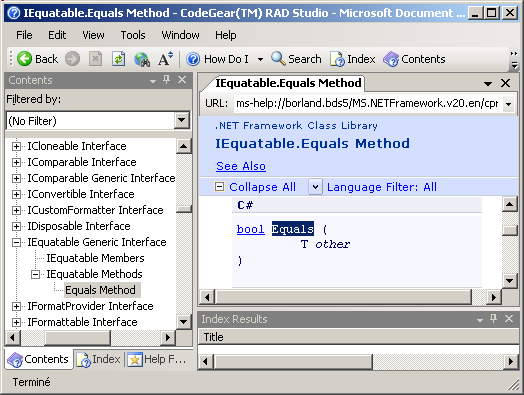Any CLASS implementing iComparable will offer a Equates() FUNCTION that we can use like this:

 IF p_c_one.Equates(p_c_two)   THEN ...ooo

The current Delphi syntax for imposing a constraint is to specify it after the type parameter identifier:

 Type c_my_class  = Class                                          // -- ...ooo... use T                                        end; // c_my_class

and for INTERFACE constraints, we simply indicate the name of which INTERFACE the concrete type arguments will implement, like this:

 Type c_my_class < T : i_my_interface < T > > = Class                                                // -- ...ooo... use T                                              end; // c_my_class

Here is a container CLASS with an ARRAY of generic values, and a f_index_of FUNCTION:

 unit u_c_genc_041_find_array;   interface     type c_find_array >=              class                m_array: Array of T_data;                m_count: Integer;                constructor create_find_array(p_length: Integer);                procedure add_to_array(p_cell: T_data);                function f_index_of(p_cell: T_data): Integer;              end; // c_find_array   implementation     // -- c_find_array     constructor c_find_array.create_find_array(p_length: Integer);       begin         Inherited Create;         SetLength(m_array, p_length);       end; // create_find_array     procedure c_find_array.add_to_array(p_cell: T_data);       begin         if m_count< Length(m_array)           then begin               writeln('  at ', m_count, ' add '+ p_cell.ToString);               m_array[m_count]:= p_cell;               Inc(m_count);             end           else Raise Exception.Create('no_more_room');       end; // add_to_array     function c_find_array.f_index_of(p_cell: T_data): Integer;       var l_index: Integer;       begin         Result:= -1;         for l_index:= 0 to m_count- 1 do         begin           if m_array[l_index].Equals(p_cell)             then begin                 Result:= l_index;                 Break;               end;         end; // for l_index       end; // f_pop   end.

When we want to use this generic CLASS, we have to specify as a type argument a TYPE which implements iEquatable. It turns out that in .Net, all the usual value types are equatable: Integer, Double, String etc.

So here is an example with Integers:

 program p_genc_041_interface_equatable;   uses SysUtils,       u_c_genc_041_find_array in 'u_c_genc_041_find_array.pas';   var g_c_integer_array: c_find_array;   begin // main     g_c_integer_array:= c_find_array.create_find_array(10);     with g_c_integer_array do     begin       add_to_array(111);       add_to_array(222);       add_to_array(333);       writeln;       writeln('index of 333 : ', f_index_of(333));       writeln('index of 777 : ', f_index_of(777));     end; // with g_c_integer_array   end. // main

• we had to repeat the type parameter in the CLASS header:

 type c_find_array >= class

The reason is that iEquatable is a "Generic Interface", as testified by the help snapshot above. For some INTERFACEs, like iComparable, there are two flavors: a generic one and a non generic one

• the Help snapshot also displays other INTERFACE which could be used in constraints, like iClonable, or iComparable which we will use in the next example.

### 5.3 - The iComparableINTERFACE constraint

To offer a generic sort CLASS, we will use separate cell and structure CLASSes. The cell contains the payload, and the structure offers the container possibility and the sort of the cells. To be able to compare two T values, we will force T to implement iComparable.

The generic CLASSes look like this:

the interesting part, of course, it the comparison of two generic values:

 If l_c_current_cell.m_data.CompareTo(l_c_current_cell.m_c_next_cell.m_data) > 0   Then

To use this generic structure, we will use a c_person CLASS with a m_first_name String. To qualify as an element which can be used in our generic structure, it has to implement the iComparable INTERFACE, and we chose to compare two persons based on their first name:

 type c_person= Class(tObject, iComparable)                  m_first_name: String;                  function CompareTo(p_c_person: c_person): Integer;                end; // c_person function c_person.CompareTo(p_c_person: c_person): Integer;   begin     if m_first_name< p_c_person.m_first_name       then Result:= -1       else Result:= + 1;;   end; // CompareTo

Here is the full code:

 program p_genc_042_i_comparable;   uses SysUtils,   u_c_genc_042_sort_linked_list in 'u_c_genc_042_sort_linked_list.pas';   // -- c_person   type c_person= Class;        c_person= Class(tObject, iComparable)                    m_first_name: String;                    Constructor create_person(p_first_name: String);                    function CompareTo(p_c_person: c_person): Integer;                    function ToString: String; Override;                  end; // c_person   constructor c_person.create_person(p_first_name: String);     begin       Inherited Create;       m_first_name:= p_first_name;     end; // createp_person   function c_person.CompareTo(p_c_person: c_person): Integer;     begin       if m_first_name< p_c_person.m_first_name         then Result:= -1         else Result:= + 1;;     end; // CompareTo   function c_person.ToString: String;     begin       Result:= m_first_name;     end; // ToString   // -- using persons   var g_c_person_sorted_list: c_linked_list;   begin // main     g_c_person_sorted_list:= c_linked_list.create_linked_list;     with g_c_person_sorted_list do     begin       add_key(c_person.create_person('aaa'));       add_key(c_person.create_person('zzz'));       add_key(c_person.create_person('mmm'));       list_generic_list;       writeln;       do_bubble_sort;       writeln;       list_generic_list;     end; // with g_c_person_sorted_list   end. // main

• the use of a linked pointed list (each cell containing a reference to the next cell) is not the most natural choice in Object Oriented Programming, with all those Collections, ArrayLists, or dynamic arrays around.
• we also must apologize for using a Bubble Sort. But for linked list, this is easier (less lines of code) to handle than using a pivot and two search values with quick sort.
• we only imposed the iComparable constraint on the type parameter of the structure, not of the basic cell.
• the generic c_cell CLASS is only a placeholder. The real CLASS is the c_person CLASS. The resulting implementation is somehow complicated, as we already mentioned: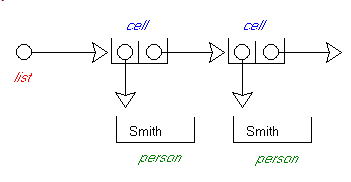• in order to be able to impose the iComparable<c_person> constraint, we had to first create a forward c_person CLASS:

 type c_person= Class;      c_person= Class(tObject, iComparable)                  m_first_name: String;                  // -- ...ooo...

• and since the constraint is on the GENERIC iComparable INTERFACE, we had to specify the <c_person> type argument when we defined the INTERFACE in the inheritance portion of the c_person CLASS:

 type c_person= Class(tObject, iComparable)

• we had also to implement the c_person.ToString FUNCTION

### 5.4 - INTERFACE constraint on a method type parameter

We can also impose an INTERFACE constraint on the type parameters of methods. Here is a CLASS with two parameterized methods:

 unit u_c_genc_043_min_max_i_comparable;   interface     uses System.Collections.Generic;     type c_min_max=              class                class function f_min >(p_one, p_two: T): T; static;                class function f_max >(p_one, p_two: T): T; static;              end; // c_min_max   implementation     // -- c_min_max     class function c_min_max.f_min(p_one, p_two: T): T;       begin         if p_one.CompareTo(p_two)< 0           then Result := p_one           else Result := p_two;       end; // f_min     class function c_min_max.f_max(p_one, p_two: T): T;       begin         if p_one.CompareTo(p_two)> 0           then Result := p_one           else Result := p_two;       end; // f_max     end. // u_c_min_max

which can be used like this:

 program p_genc_043_i_comparable;   uses SysUtils,   u_c_genc_043_min_max_i_comparable in 'u_c_genc_043_min_max_i_comparable.pas';   var g_one, g_two: Integer;       g_employee, g_salesman: String;   begin // main     g_one:= 10; g_two:= 222;     writeln('Min of ', g_one, ' and ', g_two, ' is : ',       c_min_max.f_min(g_one, g_two));     g_employee:= 'Smith'; g_salesman:= 'Allistair';     writeln('Max of ', g_employee, ' and ', g_salesman, ' is : ',       c_min_max.f_max(g_employee, g_salesman));   end. // main

Note that

• since we used CLASS FUNCTIONs, we could directly call those methods without having to create an object of type c_min_max before

### 5.5 - CONSTRUCTOR constraint

Sometime we want to be able to create an object of type T. This is helpful, for instance, when we want to create an element and add it to some structure.

We can force the type parameter to have a CONSTRUCTOR by using a constraint with the CONSTRUCTOR name:

 type c_my_class = class

CONSTRUCTOR constraints can be associated with INTERFACE constraints. In fact, we could not find an interesting example of a "pure constructor" constraint.

To demonstrate this constraint, we will present some kind of Factory Design Pattern. Imagine a product made of different parts, with different models containing the same parts but not in the same order: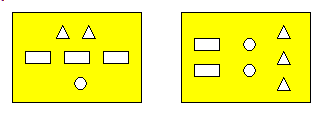To build the different makes, we can

• let the user assemble the parts whenever the model is created
• or use helper classes which contain the rules pertaining to each model
From an UML standpoint, this looks like this: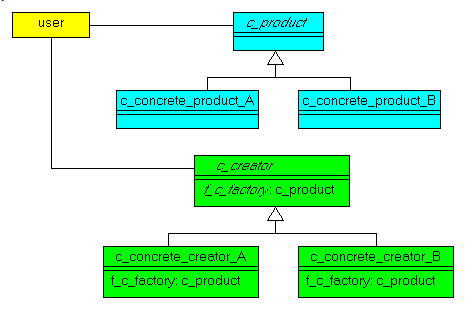Here is our factory CLASS :

 unit u_c_genc_406_factory;   interface     type c_base=            class              m_data: T_data;              procedure initialize_base(p_data: T_data); Virtual;              function ToString: String; Override;            end; // c_base          c_factory, Constructor >=            class              class function f_c_create_base(p_data: T_data): B_base;            end; // c_factory   implementation     // -- c_base     procedure c_base.initialize_base(p_data: T_data);       begin         writeln('initialize '+ Self.ClassName+ ' with '+ p_data.ToString);         m_data:= p_data;       end; // initialize_base     function c_base.ToString: String;       begin         Result:= m_data.ToString;       end; // ToString     // -- c_factory     Class Function c_factory.f_c_create_base(p_data: T_data): B_base;       begin         Result:= B_base.Create;         Result.initialize_base(p_data);       end; // create_factory     end. // u_c_factory

The important part is the last function, which create the object and calls one of its methods.

Here we use the factory to build an Integer CLASS:

 type c_integer_base=          Class( c_base )            public              m_value_1: String;              constructor Create;              procedure initialize_base(p_data: System.Int32); Override;              function ToString: String; Override;          end; // c_integer_base constructor c_integer_base.Create;   begin     Inherited;   end; // Create      procedure c_integer_base.initialize_base(p_data: System.Int32);   begin     Inherited;     m_value_1:= '111';   end; // initialize_base function c_integer_base.ToString: String;   begin     Result:= Inherited ToString+ ' '+ m_value_1.ToString;   end; // ToString // -- use the factory procedure create_integer_base;   var l_c_integer_base: c_base;   begin     l_c_integer_base:= c_factory.f_c_create_base(123);     writeln(l_c_integer_base.ToString);   end; // create_integer_base

or to build a c_person CLASS:

 type c_person=          Class            m_first_name: String;            Constructor Create;            constructor create_person(p_first_name: String);            function ToString: String; Override;          end; // c_person constructor c_person.create;   begin     Inherited Create;   end; // create constructor c_person.create_person(p_first_name: String);   begin     Inherited Create;     m_first_name:= p_first_name;   end; // create_person function c_person.ToString: String;   begin     Result:= m_first_name;   end; // ToString type c_person_base=          Class( c_base )            public              m_value_1: Double;              Constructor Create;              procedure initialize_base(p_data: c_person); Override;              function ToString: String; Override;          end; // c_person_base Constructor c_person_base.Create;   begin     Inherited;   end; // Create procedure c_person_base.initialize_base(p_data: c_person);   begin     Inherited;     m_value_1:= 3.14;   end; // initialize_base function c_person_base.ToString: String;   begin     Result:= Inherited ToString+ ' '+ m_value_1.ToString;   end; // ToString procedure create_person_base;   var l_c_person_base: c_base;   begin     l_c_person_base:= c_factory.f_c_create_base( c_person.create_person('Joe') );     writeln(l_c_person_base.ToString);   end; // create_person_base

The calls to create the objects look a little bit contrieved:

 my_c_integer_base:=      c_factory.f_c_create_base(123); my_c_person_base:=      c_factory.f_c_create_base( c_person.create_person('Joe') );

but the user who creates the object is not involved in the detail of the creation, and thats what factories are all about !

### 5.6 - iEnumerable Linked List

Lets conclude the presentation with an enumerable linked list.

In the .Net world, the containers are not based on the Win32 tList construct, but on the iEnumerable INTERFACE. This INTERFACE mainly offers the GetEnumerator FUNCTION, which returns an Enumerator with the MoveNext and Current members. A partial UML Class Diagram would be: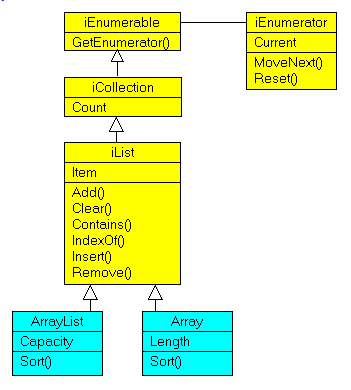As an example, the Ado.Net DataTable contains Rows which are collections of DataRows, and we can write code like:

 var my_i_row_enumerator: iEnumerator;   my_i_row_enumerator:= my_c_datatable.Rows.GetEnumerator;   while m_i_row_enumerator.MoveNext do     with m_i_row_enumearator.Current as DataRow do       // -- perform some handling on the row

Following the previous snippet, our main program will be similar to:

 program p_407_genc_ienumerable;   uses SysUtils,       System.Collections,       System.Collections.Generic,       u_c_genc_407_linked_ienumerable_list in 'u_c_genc_407_linked_ienumerable_list.pas';   procedure cg_enumerator__demo;     var l_c_cell: cg_cell;         l_cg_cell_enumerator: cig_cell_enumerator;     begin       l_c_cell:= cg_cell.create_cell('aaa', Nil);       l_c_cell:= cg_cell.create_cell('bbb', l_c_cell);       l_c_cell:= cg_cell.create_cell('ccc', l_c_cell);       l_cg_cell_enumerator:= cig_cell_enumerator.create_cell_enumerator(l_c_cell);       with l_cg_cell_enumerator do         while MoveNext do           writeln(Current.m_first_name.ToString);     end; // cg_enumerator__demo   begin // main     cg_enumerator__demo;   end. // main

To implement our list, we created the following CLASSes:

• the basic c_cell is without surprise:

 type cg_cell =          class(System.Object)            m_first_name: T;            m_c_next_cell: cg_cell;            constructor create_cell(p_first_name: T; p_c_next_cell: cg_cell);          end; // c_find_list

• the linked list will use this cell, and add the enumeration capability:

 type cg_list >=          class(System.Object, iEnumerable > )            private              m_c_first_cell: cg_cell;            public              constructor create_list;              function GetEnumerator: iEnumerator;              // -- is private              function getEnumeratorT: iEnumerator >;              function iEnumerable >.GetEnumerator = GetEnumeratorT;          end; // c_find_list

The GetEnumerator FUNCTION is obvious. But since .Net has a iEnumerable INTERFACE (in System.Collections) but also a "generic" iEnumerable INTERFACE (in System.Collections.Generic), when we implement the iEnumerable<T> INTERFACE in a CLASS we must implement both the generic and the non generic versions. This is done the two last FUNCTIONs of the CLASS.

• our enumerator is here:

 type cig_cell_enumerator=          class(tObject, iEnumerator< cg_cell >, iDisposable)              private                m_c_current_cell: cg_cell;                m_c_previous_cell: cg_cell;              strict private                function IEnumerator.get_Current = get_CurrentObject;                function get_CurrentObject: TObject;              public                function get_Current: cg_cell;                constructor create_cell_enumerator(p_c_first_cell: cg_cell );                function MoveNext: Boolean;                procedure Reset;                procedure Dispose; virtual;                property Current: cg_cell read get_Current;            end; // cig_cell_enumerator

The complete code of our iEnumerable list is:

 unit u_c_genc_407_linked_ienumerable_list;   interface     uses System.Collections, System.Collections.Generic; type cg_cell =          class(System.Object)            m_first_name: T;            m_c_next_cell: cg_cell;            constructor create_cell(p_first_name: T; p_c_next_cell: cg_cell);          end; // c_find_list      cig_cell_enumerator=          class(tObject, iEnumerator< cg_cell >, iDisposable)              private                m_c_current_cell: cg_cell;                m_c_previous_cell: cg_cell;              strict private                function IEnumerator.get_Current = get_CurrentObject;                function get_CurrentObject: TObject;              public                function get_Current: cg_cell;                constructor create_cell_enumerator(p_c_first_cell: cg_cell );                function MoveNext: Boolean;                procedure Reset;                procedure Dispose; virtual;                property Current: cg_cell read get_Current;            end; // cig_cell_enumerator      cg_list >=          class(System.Object, iEnumerable > )            private              m_c_first_cell: cg_cell;            public              constructor create_list;              function GetEnumerator: iEnumerator;              // -- is private              function getEnumeratorT: iEnumerator >;              function iEnumerable >.GetEnumerator = GetEnumeratorT;          end; // c_find_list   implementation     // -- cg_cell     constructor cg_cell.create_cell(p_first_name: T; p_c_next_cell: cg_cell);       begin         Inherited Create;         m_first_name:= p_first_name;         m_c_next_cell:= p_c_next_cell;       end; // create_cell     // -- cig_cell_enumerator     constructor cig_cell_enumerator.create_cell_enumerator(p_c_first_cell: cg_cell );       begin         Inherited Create;         m_c_current_cell:= p_c_first_cell;       end; // create_cell_enumerator     function cig_cell_enumerator.Get_Current: cg_cell;       begin         Result:= m_c_previous_cell;       end; // GetCurrent     function cig_cell_enumerator.get_CurrentObject: TObject;       begin         raise NotSupportedException.Create;       end; // get_CurrentObject     function cig_cell_enumerator.MoveNext: Boolean;       begin         m_c_previous_cell:= m_c_current_cell;         Result:= m_c_previous_cell<> Nil;         if Result           then m_c_current_cell:= m_c_current_cell.m_c_next_cell       end; // MoveNext     procedure cig_cell_enumerator.Reset;       begin         raise NotSupportedException.Create;       end; // reset     procedure cig_cell_enumerator.Dispose;       begin         raise NotSupportedException.Create;       end; // Dispose     // --  cg_list     constructor cg_list.create_list;       begin         Inherited Create;       end; // create_list     function cg_list.GetEnumerator: iEnumerator;       begin         raise NotSupportedException.Create;       end; // GetEnumerator     function cg_list.getEnumeratorT: iEnumerator >;       var l_cig_cell_enumerator: cig_cell_enumerator;       begin         l_cig_cell_enumerator:= cig_cell_enumerator.create_cell_enumerator(m_c_first_cell);       end; // getEnumeratorT   end.

### 6.1 - Generics Perspective

Generics are not a new concept. Languages like CLU already had generics. More close to Pascal, the Eiffel language had generics, and the Oberon language (Niklaus WIRTH's successor to Pascal and Modula) included them. The C++ language has the STL (Standard Template Library). And generics were introduced in the Java world (which was heavily inspired by Oberon), and from there into C#. Even UML did add a notation for type parameter !

All those versions of course have not the same functionality and implementation, but the underlying purpose is the same.

### 6.2 - What's missing

This presentation is not exhaustive. We did not present
• the DEFAULT value of a generic type
• nested CLASSes using generics
• the full syntax diagram

### 6.3 - Libraries

Among the libraries that can be found using Google:
• the C++ Stl (Standard Template Library). The Oberon developers stressed over and over again that the C++ style is only a pre-processor trick, and not a bona-fide compiled generic implementation.
• in the .Net world:
• the basic FCL (Framework Class Library) includes Queue<T>, ArrayList<T>, Dictionary<K, B>, LinkedList<V>
• there are two independent libraries : C5 and nGenerics
• Jedi certainly may implement generics, possibly deriving it from DCL (Delphi Container Library) from J.P. BEMPEL

### 6.4 - Our point of view

We are convinced that generics will be part of our daily programming tools, like Object Oriented programming. Therefore it is very important to get acquainted with them, with their strengths and weaknesses.

This is why we tried to present a diversified set of samples. To simply show some kind of generic stack or array is easy, and one could imagine the different syntactic possibilities and how the type parameter and type argument could be handled. But when we introduce several generic CLASSes, and mix genericity with inheritance, things get more involved.

But as with CLASS libraries, we only will have to dwelve into the generics intricacies if we undertake the building of some generic libraries. If this is not the case, we will simply use them. As a user it will be usefull to understand the different possibilities, some of which were presented here.

### 6.5 - What's next ?

Generics were introduced in Rad Studio 2007, since the underlying .Net 2.0 framework supports them.

The syntax has been slightly changed compared to the .Net version, which looks like (for a calculator operating on a list of values) :

 class listsCalculator       where T: new()       where C: ICalculator, new();     {       ...     }

whereas the current Delphi syntax would be like:

 type c_list_calculator         <            T_data: CONSTRUCTOR;             T_calculator: iCalculator< T_data >, CONSTRUCTOR         >         = CLASS             // -- ..ooo...           END; // c_list_calculator

It is possible that the Delphi syntax will revert to the more traditional .Net flavor when they will be implemented for the Win32 personality, sometime in 2008.

### 6.6 - No Generics Presentation at Ekon-Spring

The 24 January 2008 I received a german promotional e-mail titled "Liebe Entwickler ... Willkommen zur EKON Spring 2008 !". Der "Liber Entwickler" somehow felt maybe he could help. So, end of January, I offered to present the Genercis at this Ekon-Spring conference which was scheduled end of February. I surely was later than the "Call For Paper" deadline, but I naively assumed that when a product has 4 major innovations (dbx4, Blackfish, Asp.Net 20 and the Generics), it would not be too difficult to add an hour to the schedule, which as far as I know, did not include any Generics presentation. Well, I was badly wrong. So maybe next time, I'll try to be within deadlines, and they might consider my offer.

Meanwhile, since I still believe that Generics are a very important topic for Delphi developers, I took some of the examples I had prepared, and turned the slides into this article.

Here are the source code files:
The .ZIP file(s) contain:
• the main program (.DPROJ, .DPR, .RES)
• all units (.PAS) for units
All of the examples are .DPR console applications.

Those .ZIP

• are self-contained: you will not need any other product (unless expressly mentioned).
• will not modify your PC in any way beyond the path where you placed the .ZIP (no registry changes, no path outside from the container path creation etc).
To use the .ZIP:
• create or select any folder of your choice.
• add a ..\..\_EXE and ..\..\_EXE\DCU path
• using Delphi, compile and execute
To remove the .ZIP simply delete the folder.

The Pascal code uses the Alsacian notation, which prefixes identifier by program area: K_onstant, T_ype, G_lobal, L_ocal, P_arameter, F_unction, C_lass etc. This notation is presented in the Alsacian Notation paper.

As usual:

• we welcome any comment, criticism, enhancement, other sources or reference suggestion. Just send an e-mail to fcolibri@felix-colibri.com.
• or more simply, enter your (anonymous or with your e-mail if you want an answer) comments below and clic the "send" button
 Name : E-mail : Comments * :

## 8 - References

Here are a couple of Internet references about generics:
• Wikipedia overview of generics

• .Net generics libraries:
• for Delphi Rad Studio 2007 :
• Parameterized types : Alan BAUER - Codegear - with a nice nested CLASS example - .SWF Video, 17 Mb
• Generics : Ray KONOPKA - CodeRage II - .SWF Video, 17 MB, samples on Ray's site
• the documentation contains a chapter about generics (Rad Studio | Rad Studio (Common) | Reference | Delphi Reference | Delphi Language Guide | Generics), derived from Yooichi Tagawa draft document, which mentions "Danny's draft plan". If this is Danny THORPE, this means that the Generics were cooking since a long time, before being implemented in Rad Studio 2007.

For (visitor, factory) design patterns you may look at:

Generics are also presented in our Rad Studio 2007 trainings, and specially in object oriented trainings and as a tool for buiding business rule abstraction layers (Data Abstraction Layer) in database applications.

## 9 - The author

Felix John COLIBRI works at the Pascal Institute. Starting with Pascal in 1979, he then became involved with Object Oriented Programming, Delphi, Sql, Tcp/Ip, Html, UML. Currently, he is mainly active in the area of custom software development (new projects, maintenance, audits, BDE migration, Delphi Xe_n migrations, refactoring), Delphi Consulting and Delph training. His web site features tutorials, technical papers about programming with full downloadable source code, and the description and calendar of forthcoming Delphi, FireBird, Tcp/IP, Web Services, OOP  /  UML, Design Patterns, Unit Testing training sessions.
 Created: oct-07. Last updated: dec-2019 - 103 articles, 239 .ZIP sources, 1292 figures Contact : Felix COLIBRI - Phone: (33)1.42.83.69.36 / 06.87.88.23.91 - email:fcolibri@felix-colibri.com Copyright © Felix J. Colibri   http://www.felix-colibri.com 2004 - 2019. All rigths reserved Back:    Home  Papers  Training  Delphi developments  Links  Download### Felix J COLIBRI

+ Home
+ articles_with_sources
+ database
+ web_internet_sockets
+ rest_services
+ oop_components
– virtual_constructor
– generics_constraints
– livebindings_spelunking
+ uml_design_patterns
+ debug_and_test
+ graphic
+ controls
+ colibri_utilities
+ colibri_helpers
+ delphi
+ IDE
+ firemonkey
+ compilers
+ vcl